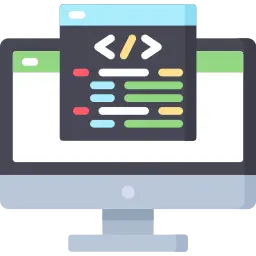# Function call by value in C

## Function Call by value Definition :

Call by value in function is passing argument method in function, in which copies of actual arguments are passed to the function.

In this method changes made to parameter inside the function does not effect the actual arguments.### Call by value Approach :

Call by value is the default method for passing arguments in C programming.
It generally indicates that a function’s code cannot change the arguments passed to the function during callbacks.

Since the value of the real parameter is copied into the formal parameter in call by value, distinct memory is allocated for the actual and formal parameters.

#### Example 1:

Run
```#include<stdio.h>
void change(int a, int b)
{
// function to chane the value

a = 100, b=200;
}
int main() {

int a = 10, b =20;
int sum =0;
sum = a+b;
printf("the sum of values before function calling : %d \n", sum);
change(a,b); // caliing the function
sum = a+b  // add again the value
printf("the sum of values after function calling : %d ", sum);
return 0;
}
```

#### Output:

```the sum of values before function calling : 30
the sum of values after function calling : 30
```

In the above example, the value of sum before and after function calling is same as arguments are passed by values and changes occur in function does not effect the actual parameters.

### Advantages of Call by value :

• Passing by value eliminates a function’s potential side effects, making your software simpler to maintain and understand.
• Reduce the likelihood of introducing subtle issues that are challenging to monitor.

### Disadvantages of Call By value :

• Copying large objects will effect the performance.
• Reallocate memory that is the same size as the object that was supplied into the function.

#### Example 2:

Run
```#include
void swap(int a, int b){ // function to swap the values

int t;
t = a;
a=b;
b=t;
}
int main() {
int a = 15, b = 50;
int sum =0;

printf("The value of a before function calling is : %d\n", a);
printf("The value of b before function calling is : %d\n", b);

swap(a,b); // calling the function

printf("The value of a after function calling is : %d\n", a);
printf("The value of b after function calling is : %d\n", b);

return 0;
}
```

#### Output:

```The value of a before function calling is : 15
The value of b before function calling is : 50
The value of a after function calling is : 15
The value of b after function calling is : 50
```

In the above  program , we write the swap function which can interchange the values, as parameters passed in  function call by value, the values don’t interchanged.

### Related Banners

Get PrepInsta Prime & get Access to all 200+ courses offered by PrepInsta in One Subscription

## Get over 200+ course One Subscription

Courses like AI/ML, Cloud Computing, Ethical Hacking, C, C++, Java, Python, DSA (All Languages), Competitive Coding (All Languages), TCS, Infosys, Wipro, Amazon, DBMS, SQL and others